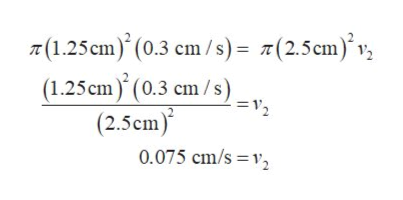# . Calculate overpressure for artery which has a puffed-up region (aneurysm) applyingBernoulli’s equation and law of continuity. Suppose, diameter of artery increases from value ?1 = 2.5cm to ?2 = 5 cm. Average flow velocity in norm is ?1 = 0.3 cm/sec.

Question
84 views

. Calculate overpressure for artery which has a puffed-up region (aneurysm) applying
Bernoulli’s equation and law of continuity. Suppose, diameter of artery increases from value ?1 = 2.5
cm to ?2 = 5 cm. Average flow velocity in norm is ?1 = 0.3 cm/sec.

check_circle

Step 1

Let the radius of the normal artery be denoted as r1 and that of the puffed up artery as r2

The radiuses r1 and r2 are known from diameters of the normal artery d1 and that of the puffed up artery d2.

Substitute 2.5 cm for d1 and 5 cm for d2 and solve for r1 and r2 as

Step 2

The equation of continuity for an incompressible fluid (Here the blood flowing in the arteries is assumed to be incompressible) is given by,

Step 3

Here, A1 is the area of cross section for the normal artery and A2 is the area of cross section for the puffed artery.v1 is the velocity of the blood in the normal artery and v2 is the velocity of blood flow in the puffed up artery.

For arteries the cross section is taken to be circular...help_outlineImage TranscriptioncloseT(1.25 cm) (0.3 cm/s)= 7(25cm) (1.25 cm (0.3 cm/s) =2 (2.5cm) 0.075 cm/s v fullscreen

### Want to see the full answer?

See Solution

#### Want to see this answer and more?

Solutions are written by subject experts who are available 24/7. Questions are typically answered within 1 hour.*

See Solution
*Response times may vary by subject and question.
Tagged in

### Fluid Mechanics